# 使用高斯混合模型建立更精确的聚类

### 介绍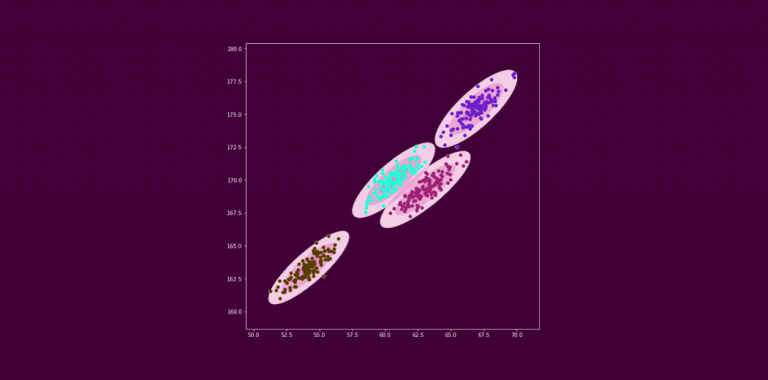### 目录

1. 聚类简介
2. k-means聚类简介
3. k-means聚类的缺点
4. 介绍高斯混合模型
5. 高斯分布
6. 期望最大化EM算法
7. 高斯混合模型的期望最大化
8. 在Python中实现用于聚类的高斯混合模型

### 聚类简介

• 赚得多，花得多
• 赚得多，花得少
• 赚得少，花得少
• 赚得少，花得多### k-means聚类简介

k-means聚类是一种基于距离的算法。这意味着它试图将最近的点分组形成一个聚类。

### k-means聚类的缺点

k-means聚类概念听起来很不错，不是吗?它易于理解，相对容易实现，并且可以应用于相当多的用例中。但也有一些我们需要注意的缺陷和限制。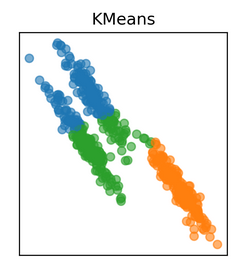### 高斯混合模型简介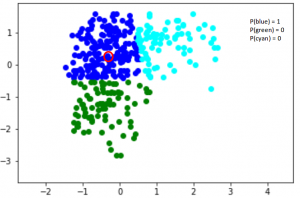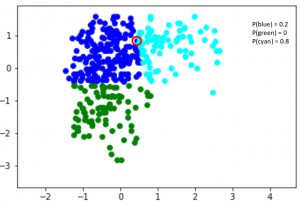### 高斯分布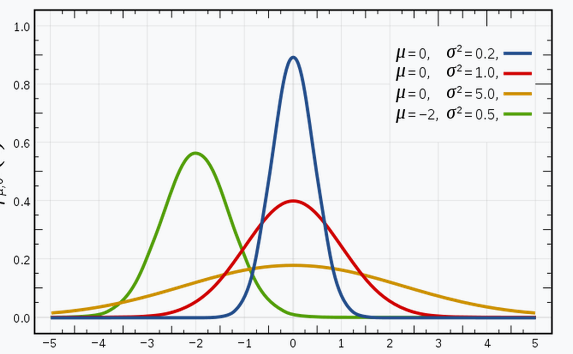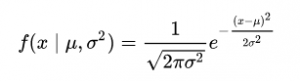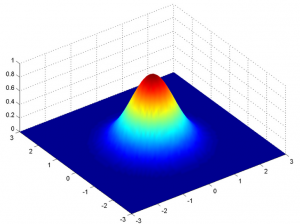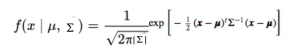### 期望最大化EM算法

• E步:在此步骤中，可用数据用于估计(猜测)缺失变量的值
• M步:根据E步生成的估计值，使用完整的数据更新参数

### 高斯混合模型的期望最大化

E步: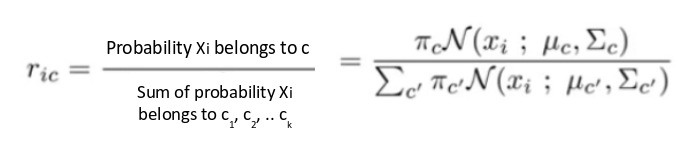M步:

E步后,我们回去更新Π,μ和Σ值。这些资料的更新方式如下:

1. 新的权重定义为簇内数据的数量与数据总数量之比: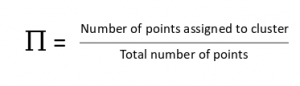1. 均值和协方差矩阵根据分配给分布的值更新，与数据点的概率值成比例。因此，一个更有可能成为该分布一部分的数据点将有更大贡献: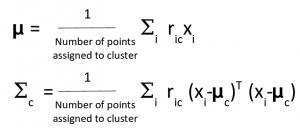k-means只考虑更新簇中心的均值，而GMM则考虑数据的均值和方差。

### 在Python中实现高斯混合模型

import pandas as pd

plt.figure(figsize=(7,7))
plt.scatter(data["Weight"],data["Height"])
plt.xlabel('Weight')
plt.ylabel('Height')
plt.title('Data Distribution')
plt.show()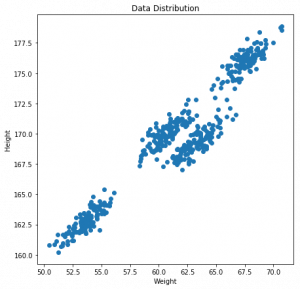#训练k-means模型
from sklearn.cluster import KMeans
kmeans = KMeans(n_clusters=4)
kmeans.fit(data)

#kmeans预测
pred = kmeans.predict(data)
frame = pd.DataFrame(data)
frame['cluster'] = pred
frame.columns = ['Weight', 'Height', 'cluster']

#输出结果
color=['blue','green','cyan', 'black']
for k in range(0,4):
data = frame[frame["cluster"]==k]
plt.scatter(data["Weight"],data["Height"],c=color[k])
plt.show()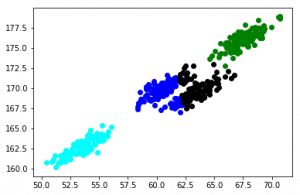import pandas as pd

# 训练高斯混合模型
from sklearn.mixture import GaussianMixture
gmm = GaussianMixture(n_components=4)
gmm.fit(data)

#GMM预测
labels = gmm.predict(data)
frame = pd.DataFrame(data)
frame['cluster'] = labels
frame.columns = ['Weight', 'Height', 'cluster']

color=['blue','green','cyan', 'black']
for k in range(0,4):
data = frame[frame["cluster"]==k]
plt.scatter(data["Weight"],data["Height"],c=color[k])
plt.show()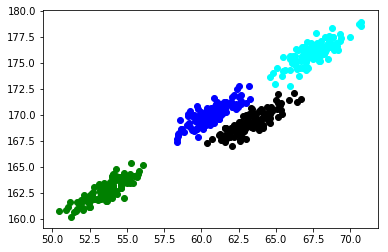### 结尾©️2019 CSDN 皮肤主题: 大白 设计师: CSDN官方博客